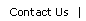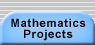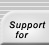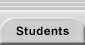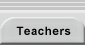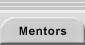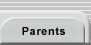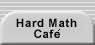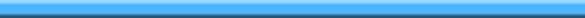# The Fundamental Theorem of Arithmetic

The fundamental theorem of arithmetic states that every positive integer can be factored in one and only one way into a product of primes.

For example, 60 can be expressed as 22.3.5. No other combination of prime factors (excluding different orderings of 2, 2, 3, and 5) will yield 60. Note that 1 is not considered prime because its inclusion as a prime number would complicate the statement of many theorems including this one. For example, if 1 were prime, then 60 could equal both 22.3.5 and 1.22.3.5.

For a proof of the fundamental theorem of arithmetic, see Euclid's Algorithm and read the entire page.Translations of mathematical formulas for web display were created by tex4ht. © Copyright 2003 Education Development Center, Inc. (EDC)